# MAFS.4.NF.2.3

Understand a fraction a/b with a > 1 as a sum of fractions 1/b.
1. Understand addition and subtraction of fractions as joining and separating parts referring to the same whole.
2. Decompose a fraction into a sum of fractions with the same denominator in more than one way, recording each decomposition by an equation. Justify decompositions, e.g., by using a visual fraction model. Examples: 3/8 = 1/8 + 1/8 + 1/8 ; 3/8 = 1/8 + 2/8 ; 2 1/8 = 1 + 1 + 1/8 = 8/8 + 8/8 + 1/8.
3. Add and subtract mixed numbers with like denominators, e.g., by replacing each mixed number with an equivalent fraction, and/or by using properties of operations and the relationship between addition and subtraction.
4. Solve word problems involving addition and subtraction of fractions referring to the same whole and having like denominators, e.g., by using visual fraction models and equations to represent the problem.

### Clarifications

Examples of Opportunities for In-Depth Focus

This standard represents an important step in the multi-grade progression for addition and subtraction of fractions. Students extend their prior understanding of addition and subtraction to add and subtract fractions with like denominators by thinking of adding or subtracting so many unit fractions.
General Information
Subject Area: Mathematics
Domain-Subdomain: Number and Operations - Fractions
Cluster: Level 2: Basic Application of Skills & Concepts
Cluster: Build fractions from unit fractions by applying and extending previous understandings of operations on whole numbers. (Major Cluster) -

Clusters should not be sorted from Major to Supporting and then taught in that order. To do so would strip the coherence of the mathematical ideas and miss the opportunity to enhance the major work of the grade with the supporting clusters.

Date of Last Rating: 02/14
Status: State Board Approved
Assessed: Yes
Test Item Specifications

• Assessment Limits :
Denominators of given fractions are limited to: 2, 3, 4, 5, 6, 8, 10, 12, 100. Mixed numbers and fractions must contain like denominators. Items must reference the same whole. Visual fraction models are limited to circular models, rectangular models, and number line models.
• Calculator :

No

• Context :

Allowable. Required for MAFS.4.NF.2.3d

Sample Test Items (5)
• Test Item #: Sample Item 1
• Question:

What is the value of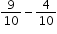?

• Difficulty: N/A
• Type: EE: Equation Editor

• Test Item #: Sample Item 2
• Question:

What is the value of the following expression?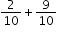• Difficulty: N/A
• Type: MC: Multiple Choice

• Test Item #: Sample Item 3
• Question:

Sue had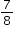of a cup of flour. She used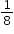of a cup.

How much flour, in cups, does Sue have left?

• Difficulty: N/A
• Type: EE: Equation Editor

• Test Item #: Sample Item 4
• Question:

What is the sum ofand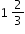?

• Difficulty: N/A
• Type: EE: Equation Editor

• Test Item #: Sample Item 5
• Question:

Select all the equations that show different ways to represent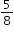.

• Difficulty: N/A
• Type: MS: Multiselect

## Related Courses

This benchmark is part of these courses.
5012060: Mathematics - Grade Four (Specifically in versions: 2014 - 2015, 2015 - 2022 (current), 2022 and beyond)
7712050: Access Mathematics Grade 4 (Specifically in versions: 2014 - 2015, 2015 - 2018, 2018 - 2022 (current), 2022 and beyond)
5012065: Grade 4 Accelerated Mathematics (Specifically in versions: 2019 - 2022 (current), 2022 and beyond)
5012015: Foundational Skills in Mathematics 3-5 (Specifically in versions: 2019 - 2022 (current), 2022 and beyond)

## Related Access Points

Alternate version of this benchmark for students with significant cognitive disabilities.
MAFS.4.NF.2.AP.3a: Using a representation, decompose a fraction into multiple copies of a unit fraction (e.g., 3/4 = 1/4 + 1/4+ 1/4).
MAFS.4.NF.2.AP.3b: Add and subtract fractions with like denominators (2, 3, 4 or 8) using representations.
MAFS.4.NF.2.AP.3c: Solve word problems involving addition and subtraction of fractions with like denominators (2, 3, 4 or 8).

## Related Resources

Vetted resources educators can use to teach the concepts and skills in this benchmark.

## Assessments

Sample 4 - Fourth Grade Math State Interim Assessment:

This is a State Interim Assessment for fourth grade.

Type: Assessment

Sample 3 - Fourth Grade Math State Interim Assessment:

This is a State Interim Assessment for fourth grade.

Type: Assessment

Sample 2 - Fourth Grade Math State Interim Assessment:

This is a State Interim Assessment for fourth grade.

Type: Assessment

Sample 1 - Fourth Grade Math State Interim Assessment:

This is a State Interim Assessment for fourth grade.

Type: Assessment

## Educational Game

Fraction Quiz:

Test your fraction skills by answering questions on this site. This quiz asks you to simplify fractions, convert fractions to decimals and percentages, and answer algebra questions involving fractions. You can even choose difficulty level, question types, and time limit.

Type: Educational Game

## Formative Assessments

Students are given pairs of mixed numbers to either add or subtract.

Type: Formative Assessment

Fraction Word Problems:

Students are asked to solve a word problem that involves subtracting fractions with like denominators. Students then analyze a word problem involving subtraction of unlike unit quantities.

Type: Formative Assessment

Decomposing Three-Fifths:

Students are asked to use a visual fraction model to decompose three-fifths in two different ways.

Type: Formative Assessment

Anna Marie and the Pizza:

Students are asked to solve a word problem that involves adding fractions with like denominators. Students then analyze a word problem involving addition of unlike unit quantities.

Type: Formative Assessment

## Lesson Plans

Relay Races:

In this lesson, students solve word problems related to races to determine addends of fractions with like denominators that sum to a fraction that is less than or equal to one and has the same denominator as the addends. The focus is on addition, decomposing a fraction into a sum of fractions in more than one way, drawing linear models, and writing equations to represent the problems.

Type: Lesson Plan

Learning to Love Like Denominators:

Students engage in problem solving to explore the addition and subtraction of fractions with like denominators. Students make sense of the structure of addition and subtraction equations with like denominators and make generalizations to move from using manipulatives, pictures and number lines to simply adding or subtracting the numerator.

Type: Lesson Plan

Adding and Subtracting in the Real World with Unit Fractions:

Students will use unit fractions, and counting on or back by unit fractions, to solve addition and subtraction real world problems.

Type: Lesson Plan

Looking for Patterns in a Sequence of Fractions:

Students generate and describe a numerical pattern using the multiplication and subtraction of fractions.

Type: Lesson Plan

Decomposing Fractions:

Using circle fraction manipulative, students will decompose fractions to discover adding fractions with like denominators.

Type: Lesson Plan

Marshmallow Math:

In this lesson, students are physically engaged in measuring distances of tossed marshmallows to the nearest 1/2 foot. Using their measurements, they will represent the data on a line plot and then solve word problems involving addition and subtraction of mixed numbers. This is a fun lesson that motivates students to become excited about the difficult world of fractions.

Type: Lesson Plan

## Original Student Tutorial

The Leftover Dessert Dilemma:

Learn how to decompose a fraction into a sum of fractions with common denominators with this interactive tutorial.

Type: Original Student Tutorial

Making 22 Seventeenths in Different Ways:

This task is a straightforward task related to adding fractions with the same denominator. The main purpose is to emphasize that there are many ways to decompose a fraction as a sum of fractions.

Comparing two different pizzas:

The focus of this task is on understanding that fractions, in an explicit context, are fractions of a specific whole. In this this problem there are three different wholes: the medium pizza, the large pizza, and the two pizzas taken together. This task is best suited for instruction. Students can practice explaining their reasoning to each other in pairs or as part of a whole group discussion.

Writing a Mixed Number as an Equivalent Fraction:

The purpose of this task is to help students understand and articulate the reasons for the steps in the usual algorithm for converting a mixed number into an equivalent fraction. Step two shows that the algorithm is merely a shortcut for finding a common denominator between two fractions. This concept is an important precursor to adding mixed numbers and fractions with like denominators and as such, step two should be a point of emphasis. This task is appropriate for either instruction or formative assessment.

Peaches:

This task provides a context where it is appropriate for students to subtract fractions with a common denominator; it could be used for either assessment or instructional purposes. For this particular task, teachers should anticipate two types of solution approaches: one where students subtract the whole numbers and the fractions separately and one where students convert the mixed numbers to improper fractions and then proceed to subtract.

Plastic Building Blocks:

The purpose of this task is to have students add mixed numbers with like denominators. This task illustrates the different kinds of solution approaches students might take to such a task. Two general approaches should be anticipated: one where students calculate exactly how many buckets of blocks the boys have to determine an answer, and one where students compare the given numbers to benchmark numbers.

## Tutorials

What Fraction of Spider Eyes are Looking at Me?:

This Khan Academy video uses authentic pictures to present addition of two fractions with common denominators.

Type: Tutorial

Figuring Out How Much of a Pizza is Left:

This Khan Academy video solves two word problems using visual fraction models.

Type: Tutorial

Comparing Fractions:

This tutorial for student audiences will assist learners with a further understanding that fractions are a way of showing part of a whole. Yet some fractions are larger than others. So this tutorial will help to refresh the understanding for the comparison of fractions. Students will be able to navigate the teaching portion of the tutorial at their own pace and test their understanding after each step of the lesson with a "Try This" section. The "Try This" section will monitor students answers and self-check by a right answer turning orange and a wrong answer dissolving.

Type: Tutorial

In this web-based tutorial, students learn procedures for adding fractions with like and unlike denominators. The tutorial includes visual representations of the problems using pizzas, animations of the algorithm, and links to related lessons, worksheets, and practice problems.

Type: Tutorial

## Virtual Manipulative

Fraction Game:

This virtual manipulative allows individual students to work with fraction relationships. (There is also a link to a two-player version.)

Type: Virtual Manipulative

## MFAS Formative Assessments

Students are given pairs of mixed numbers to either add or subtract.

Anna Marie and the Pizza:

Students are asked to solve a word problem that involves adding fractions with like denominators. Students then analyze a word problem involving addition of unlike unit quantities.

Decomposing Three-Fifths:

Students are asked to use a visual fraction model to decompose three-fifths in two different ways.

Fraction Word Problems:

Students are asked to solve a word problem that involves subtracting fractions with like denominators. Students then analyze a word problem involving subtraction of unlike unit quantities.

## Original Student Tutorials Mathematics - Grades K-5

The Leftover Dessert Dilemma:

Learn how to decompose a fraction into a sum of fractions with common denominators with this interactive tutorial.

## Student Resources

Vetted resources students can use to learn the concepts and skills in this benchmark.

## Original Student Tutorial

The Leftover Dessert Dilemma:

Learn how to decompose a fraction into a sum of fractions with common denominators with this interactive tutorial.

Type: Original Student Tutorial

## Educational Game

Fraction Quiz:

Test your fraction skills by answering questions on this site. This quiz asks you to simplify fractions, convert fractions to decimals and percentages, and answer algebra questions involving fractions. You can even choose difficulty level, question types, and time limit.

Type: Educational Game

Making 22 Seventeenths in Different Ways:

This task is a straightforward task related to adding fractions with the same denominator. The main purpose is to emphasize that there are many ways to decompose a fraction as a sum of fractions.

Comparing two different pizzas:

The focus of this task is on understanding that fractions, in an explicit context, are fractions of a specific whole. In this this problem there are three different wholes: the medium pizza, the large pizza, and the two pizzas taken together. This task is best suited for instruction. Students can practice explaining their reasoning to each other in pairs or as part of a whole group discussion.

Writing a Mixed Number as an Equivalent Fraction:

The purpose of this task is to help students understand and articulate the reasons for the steps in the usual algorithm for converting a mixed number into an equivalent fraction. Step two shows that the algorithm is merely a shortcut for finding a common denominator between two fractions. This concept is an important precursor to adding mixed numbers and fractions with like denominators and as such, step two should be a point of emphasis. This task is appropriate for either instruction or formative assessment.

Peaches:

This task provides a context where it is appropriate for students to subtract fractions with a common denominator; it could be used for either assessment or instructional purposes. For this particular task, teachers should anticipate two types of solution approaches: one where students subtract the whole numbers and the fractions separately and one where students convert the mixed numbers to improper fractions and then proceed to subtract.

Plastic Building Blocks:

The purpose of this task is to have students add mixed numbers with like denominators. This task illustrates the different kinds of solution approaches students might take to such a task. Two general approaches should be anticipated: one where students calculate exactly how many buckets of blocks the boys have to determine an answer, and one where students compare the given numbers to benchmark numbers.

## Tutorials

What Fraction of Spider Eyes are Looking at Me?:

This Khan Academy video uses authentic pictures to present addition of two fractions with common denominators.

Type: Tutorial

Figuring Out How Much of a Pizza is Left:

This Khan Academy video solves two word problems using visual fraction models.

Type: Tutorial

Comparing Fractions:

This tutorial for student audiences will assist learners with a further understanding that fractions are a way of showing part of a whole. Yet some fractions are larger than others. So this tutorial will help to refresh the understanding for the comparison of fractions. Students will be able to navigate the teaching portion of the tutorial at their own pace and test their understanding after each step of the lesson with a "Try This" section. The "Try This" section will monitor students answers and self-check by a right answer turning orange and a wrong answer dissolving.

Type: Tutorial

## Virtual Manipulative

Fraction Game:

This virtual manipulative allows individual students to work with fraction relationships. (There is also a link to a two-player version.)

Type: Virtual Manipulative

## Parent Resources

Vetted resources caregivers can use to help students learn the concepts and skills in this benchmark.

Making 22 Seventeenths in Different Ways:

This task is a straightforward task related to adding fractions with the same denominator. The main purpose is to emphasize that there are many ways to decompose a fraction as a sum of fractions.

Comparing two different pizzas:

The focus of this task is on understanding that fractions, in an explicit context, are fractions of a specific whole. In this this problem there are three different wholes: the medium pizza, the large pizza, and the two pizzas taken together. This task is best suited for instruction. Students can practice explaining their reasoning to each other in pairs or as part of a whole group discussion.

Writing a Mixed Number as an Equivalent Fraction:

The purpose of this task is to help students understand and articulate the reasons for the steps in the usual algorithm for converting a mixed number into an equivalent fraction. Step two shows that the algorithm is merely a shortcut for finding a common denominator between two fractions. This concept is an important precursor to adding mixed numbers and fractions with like denominators and as such, step two should be a point of emphasis. This task is appropriate for either instruction or formative assessment.

Peaches:

This task provides a context where it is appropriate for students to subtract fractions with a common denominator; it could be used for either assessment or instructional purposes. For this particular task, teachers should anticipate two types of solution approaches: one where students subtract the whole numbers and the fractions separately and one where students convert the mixed numbers to improper fractions and then proceed to subtract.

Plastic Building Blocks:

The purpose of this task is to have students add mixed numbers with like denominators. This task illustrates the different kinds of solution approaches students might take to such a task. Two general approaches should be anticipated: one where students calculate exactly how many buckets of blocks the boys have to determine an answer, and one where students compare the given numbers to benchmark numbers.